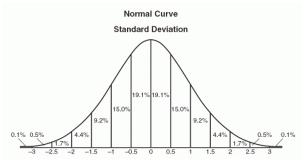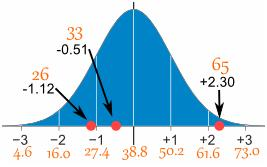# Statistics - Normal Distribution

A normal distribution is an arrangement of a data set in which most values cluster in the middle of the range and the rest taper off symmetrically toward either extreme. Height is one simple example of something that follows a normal distribution pattern: Most people are of average height the numbers of people that are taller and shorter than average are fairly equal and a very small (and still roughly equivalent) number of people are either extremely tall or extremely short.Here's an example of a normal distribution curve:A graphical representation of a normal distribution is sometimes called a bell curve because of its flared shape. The precise shape can vary according to the distribution of the population but the peak is always in the middle and the curve is always symmetrical. In a normal distribution the mean mode and median are all the same.

## Formula

${y = \frac{1}{\sqrt {2 \pi}}e^{\frac{-(x - \mu)^2}{2 \sigma}} }$

Where −

• ${\mu}$ = Mean

• ${\sigma}$ = Standard Deviation

• ${\pi \approx 3.14159}$

• ${e \approx 2.71828}$

### Example

Problem Statement:

A survey of daily travel time had these results (in minutes):

 26 33 65 28 34 55 25 44 50 36 26 37 43 62 35 38 45 32 28 34

The Mean is 38.8 minutes, and the Standard Deviation is 11.4 minutes. Convert the values to z - scores and prepare the Normal Distribution Graph.

Solution:

The formula for z-score that we have been using:

${z = \frac{x - \mu}{\sigma} }$

Where −

• ${z}$ = the "z-score" (Standard Score)

• ${x}$ = the value to be standardized

• ${\mu}$ = mean

• ${\sigma}$ = the standard deviation

To convert 26:

First subtract the mean: 26-38.8 = -12.8,

Then divide by the Standard Deviation: -12.8/11.4 = -1.12

So 26 is -1.12 Standard Deviation from the Mean

Here are the first three conversions.

Original ValueCalculationStandard Score (z-score)
26(26-38.8) / 11.4 =-1.12
33(33-38.8) / 11.4 =-0.51
65(65-38.8) / 11.4 =-2.30
.........

And here they graphically represent: